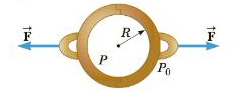Chapter 9, Problem 89AP

Chapter
Section
Textbook Problem

In about 1657. Otto von Guericke, inventor of the air pump, evacuated a sphere made of two brass hemispheres (Fig. P9.89). Two teams of eight horses each could pull the hemispheres apart only on some trials and then “with greatest difficulty,” with the resulting sound likened to a cannon firing. Find the force F required to pull the thin-walled evacuated hemispheres apart in terms of R, the radius of the hemispheres, P the pressure inside the hemispheres, and atmospheric pressure P0.Figure P9.89

To determine
The force required to pull the thin-walled evacuated hemispheres apart.

Explanation

The effective area is the area projected on the plane by sphere which is equal to the cross-sectional area of the sphere and the gauge pressure is Pgauge=P0P .

The pressure that acts on the surface of coupled hemispheres would be radially inward on each point on it and it means that forces acting on each surface element of the hemispheres to hold it together.

Thus, force on each side that applied horizontal direction might be equal to that of the net force on the effective area called the cross-sectional area of the sphere

Still sussing out bartleby?

Check out a sample textbook solution.

See a sample solution

The Solution to Your Study Problems

Bartleby provides explanations to thousands of textbook problems written by our experts, many with advanced degrees!

Get Started

Find more solutions based on key concepts